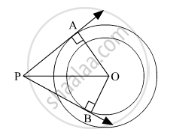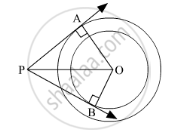Share
Notifications

View all notifications
Books Shortlist
Your shortlist is empty

# In Fig. 3 Are Two Concentric Circles of Radii 6 Cm and 4 Cm with Centre O. If Ap is a Tangent to the Larger Circle and Bp to the Smaller Circle and Length of Ap is 8 Cm, Find the Length of Bp ? - CBSE Class 10 - Mathematics

Login
Create free account

Forgot password?
ConceptCircles Examples and Solutions

#### Question

In fig. 3 are two concentric circles of radii 6 cm and 4 cm with centre O. If AP is a tangent to the larger circle and BP to the smaller circle and length of AP is 8 cm, find the length of BP ?#### SolutionGiven:
OA = 6 cm
OB = 4 cm
AP = 8 cm

Consider ∆OAP.
By Pythagoras' theorem, we have
OA2 + AP2 = PO2
6+ 8= PO2
⇒ PO2 = 100
⇒ PO = 10 cm

Now, consider ∆OBP.
By Pythagoras' theorem, we have
OB2 + BP2 = PO2
⇒ 4+ BP2 = 102
⇒ BP2 = 84
⇒ BP =

$2\sqrt{21}$cm

Hence, the length of BP is

$2\sqrt{21}$cm.
Is there an error in this question or solution?

#### Video TutorialsVIEW ALL 

Solution In Fig. 3 Are Two Concentric Circles of Radii 6 Cm and 4 Cm with Centre O. If Ap is a Tangent to the Larger Circle and Bp to the Smaller Circle and Length of Ap is 8 Cm, Find the Length of Bp ? Concept: Circles Examples and Solutions.
S